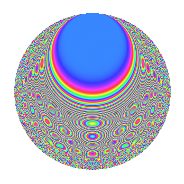# Properties

 Label 1336.2.hLevel 1336 Weight 2 Character orbit h Rep. character $$\chi_{1336}(667,\cdot)$$ Character field $$\Q$$ Dimension 166 Sturm bound 336

# Related objects

## Defining parameters

 Level: $$N$$ $$=$$ $$1336 = 2^{3} \cdot 167$$ Weight: $$k$$ $$=$$ $$2$$ Character orbit: $$[\chi]$$ $$=$$ 1336.h (of order $$2$$ and degree $$1$$) Character conductor: $$\operatorname{cond}(\chi)$$ $$=$$ $$1336$$ Character field: $$\Q$$ Sturm bound: $$336$$

## Dimensions

The following table gives the dimensions of various subspaces of $$M_{2}(1336, [\chi])$$.

Total New Old
Modular forms 170 170 0
Cusp forms 166 166 0
Eisenstein series 4 4 0

## Trace form

 $$166q - 2q^{2} - 4q^{3} - 6q^{4} - 8q^{6} + 4q^{8} + 158q^{9} + O(q^{10})$$ $$166q - 2q^{2} - 4q^{3} - 6q^{4} - 8q^{6} + 4q^{8} + 158q^{9} - 4q^{11} + 6q^{12} + 10q^{14} - 22q^{16} + 8q^{18} - 20q^{19} - 8q^{22} + 26q^{24} + 154q^{25} + 8q^{27} + 32q^{28} - 42q^{32} - 16q^{33} - 54q^{36} - 4q^{38} + 17q^{42} + 11q^{44} - 23q^{48} - 158q^{49} + 8q^{50} - 21q^{54} + 14q^{56} - 16q^{57} + 14q^{58} - 15q^{62} - 48q^{64} - 24q^{65} - 28q^{66} - 25q^{72} - 60q^{75} + 118q^{81} + 61q^{84} - 4q^{88} + 12q^{89} - 18q^{94} + 26q^{96} - 4q^{97} + 9q^{98} + 12q^{99} + O(q^{100})$$

## Decomposition of $$S_{2}^{\mathrm{new}}(1336, [\chi])$$ into newform subspaces

The newforms in this space have not yet been added to the LMFDB.

## Hecke characteristic polynomials

There are no characteristic polynomials of Hecke operators in the database